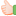1. ## Simple Puzzle...

Solve the following equations:

T-W-O=2
(T/W)/O=2

where T, W, and O are single-digit integers and they are distinct integers.Reply With Quote

2. ## Re: Simple Puzzle...

Solving this puzzle I get O=1, T=6 and W=3
Kindly update whether I have solved it correctly.Reply With Quote

3. ## Re: Simple Puzzle...

-- JamesReply With Quote

4. ##Re: Simple Puzzle...

First of all looking at the second condition it is clear that O can be either 1,2 or 4.
Thus when O is 1 we can have

T=6, W=3 (through condition 2 only) -------------(1 )
T=4,W=2 ------------------------------(2)
T=8,W=4 ----------------------------(3 )

Now looking at 1,2,3 we can see (1) satisfies the first condition T-W-O=2 .
Thus we are not suppose to check for O=2 and 4

And thus our answer is T=6,W=3,O=1Reply With Quote

####Posting Permissions

• You may not post new threads
• You may not post replies
• You may not post attachments
• You may not edit your posts
•